# Investigating Euler's Polyhedral Formula Using Original Sources

Author(s):

Teachers of mathematics would like to motivate their students. Approaches to achieve motivation can be divided into three categories:

• You can use the present. How does a topic relate to material recently covered in the course?
• You can use the future. After learning a topic, you will be prepared to apply your knowledge and solve the many real-world problems you will undoubtedly encounter in the future.
• You can use the past. What is the historical context of a given topic? Why did anyone consider it in the first place? What was the original formulation and presentation like?

Let us assume that we wish to use the past.

Who should we study? If we are interested in examining original sources, a natural choice is Leonhard Euler. He is a master of exposition; he writes to be understood; he doesn't assume extensive background; he uses lots of examples; ideas gradually build on each other, and so on. In short, Euler writes in precisely the style that many of us hope to achieve when we teach.

What should we read? In the case of Euler, there are literally hundreds of things from which to choose! Perhaps we would like to choose a topic that is particularly "fun"; one that does not involve advanced topics such as calculus or mechanics. Even with this restriction, there is a variety of material available. Possible topics include: the bridges of Königsberg and the beginnings of graph theory, the 36 officers problem and Latin squares, knight's tours on a chessboard, investigating Fermat numbers, and many more. Here, we will discuss Euler's first paper on polyhedra. This topic is particularly appealing to a large audience of students because it is very visual. Particularly in mathematics, students tend to appreciate objects they can visualize and even manipulate—many students prefer the concrete (such as models you can construct) to the abstract (such as manipulations of functions and equations). However, there are plenty of functions and equations to be found in this topic; they are potentially easier to grasp because they relate to objects readily visualized.

For the Euler enthusiast, the particular paper described below is Elementa Doctrinae Solidorum, Eneström number 230 [Euler 1758].

Where can this paper be used? This topic is appropriate for a course in Discrete Mathematics, Graph Theory, History of Mathematics, or perhaps even a general course in mathematics for an audience of Liberal Arts students.

How can this paper be used and this module be effectively implemented? The basic material required is all available online. The Euler Archive (located at http://eulerarchive.maa.org/) has scanned versions of Euler's original publications freely available for download. The publication under discussion here may be downloaded in its entirety from the Euler Archive or via this link. In some cases, the Archive also includes links to translations and lists of references. The other major resource is a monthly column, How Euler Did It, written by Dr. Edward Sandifer, originally published at MAA Online and now available from the Euler Archive at http://eulerarchive.maa.org/hedi/index.html. The June 2004 and July 2004 columns also address the work of Euler discussed in this article; we will avoid overlapping the material discussed in these columns as much as possible. In addition to on-line resources, instructors may wish to compare and contrast Euler's results and presentation style with that of a modern textbook, such as [West 2001]. Furthermore, depending on the time available for this topic and the level of the course, [Lakatos 1976] is an excellent companion book that discusses Euler's polyhedral formula in depth.

This article concerns using Euler's original paper to investigate properties of polyhedra, in particular, convincing the reader that it is a worthwhile approach to take.

# Investigating Euler's Polyhedral Formula Using Original Sources - Original Sources: Who, What, Where, How

Author(s):

Teachers of mathematics would like to motivate their students. Approaches to achieve motivation can be divided into three categories:

• You can use the present. How does a topic relate to material recently covered in the course?
• You can use the future. After learning a topic, you will be prepared to apply your knowledge and solve the many real-world problems you will undoubtedly encounter in the future.
• You can use the past. What is the historical context of a given topic? Why did anyone consider it in the first place? What was the original formulation and presentation like?

Let us assume that we wish to use the past.

Who should we study? If we are interested in examining original sources, a natural choice is Leonhard Euler. He is a master of exposition; he writes to be understood; he doesn't assume extensive background; he uses lots of examples; ideas gradually build on each other, and so on. In short, Euler writes in precisely the style that many of us hope to achieve when we teach.

What should we read? In the case of Euler, there are literally hundreds of things from which to choose! Perhaps we would like to choose a topic that is particularly "fun"; one that does not involve advanced topics such as calculus or mechanics. Even with this restriction, there is a variety of material available. Possible topics include: the bridges of Königsberg and the beginnings of graph theory, the 36 officers problem and Latin squares, knight's tours on a chessboard, investigating Fermat numbers, and many more. Here, we will discuss Euler's first paper on polyhedra. This topic is particularly appealing to a large audience of students because it is very visual. Particularly in mathematics, students tend to appreciate objects they can visualize and even manipulate—many students prefer the concrete (such as models you can construct) to the abstract (such as manipulations of functions and equations). However, there are plenty of functions and equations to be found in this topic; they are potentially easier to grasp because they relate to objects readily visualized.

For the Euler enthusiast, the particular paper described below is Elementa Doctrinae Solidorum, Eneström number 230 [Euler 1758].

Where can this paper be used? This topic is appropriate for a course in Discrete Mathematics, Graph Theory, History of Mathematics, or perhaps even a general course in mathematics for an audience of Liberal Arts students.

How can this paper be used and this module be effectively implemented? The basic material required is all available online. The Euler Archive (located at http://eulerarchive.maa.org/) has scanned versions of Euler's original publications freely available for download. The publication under discussion here may be downloaded in its entirety from the Euler Archive or via this link. In some cases, the Archive also includes links to translations and lists of references. The other major resource is a monthly column, How Euler Did It, written by Dr. Edward Sandifer, originally published at MAA Online and now available from the Euler Archive at http://eulerarchive.maa.org/hedi/index.html. The June 2004 and July 2004 columns also address the work of Euler discussed in this article; we will avoid overlapping the material discussed in these columns as much as possible. In addition to on-line resources, instructors may wish to compare and contrast Euler's results and presentation style with that of a modern textbook, such as [West 2001]. Furthermore, depending on the time available for this topic and the level of the course, [Lakatos 1976] is an excellent companion book that discusses Euler's polyhedral formula in depth.

This article concerns using Euler's original paper to investigate properties of polyhedra, in particular, convincing the reader that it is a worthwhile approach to take.

# Investigating Euler's Polyhedral Formula Using Original Sources - Understanding Latin

Author(s):

At first glance, one may be discouraged because the article is written in Latin. However, we may take heart, remembering that Euler took great pains in writing to be understood. In particular, Latin was nobody's first language in the 18th century. Conscientious of this fact, Euler used straightforward vocabulary and linguistic constructions in his writing; the prose is not flowery. Three pieces of advice for the Latin amateur (from a fellow Latin amateur) are:

1. Most sections will require multiple readings. During a first reading, aim for a general idea of what is being discussed by looking at the roots of words. (Many of the words we use today have their roots in Latin; many examples will follow throughout the remainder of this article.) Do not get hung up on the tenses of words, on the distinction between adjectives and adverbs, and so forth. If the goal was to write a translation, then these details would be important, but we shall assume that the goal is to gain an understanding of the results and an appreciation of the style in which they were originally presented.

2. Do not be misled by the typesetting. Often times our letter "s" appears to be an "f", while the letter "i" is used in place of a "j", or a "u" in place of a "v". This is not always the case, but it does happen sometimes. If a word looks unfamiliar, trying a letter swap will sometimes reveal a word or root of a word that is familiar to us, allow us to glean an idea of what is being said. A few examples that appear repeatedly in this paper include the Latin words folidi (read as solidi), maior (read as major), and conuexium (read as convexium). Often, looking at the context will help.

3. Always keep a Latin-to-English dictionary handy. The author prefers the on-line dictionary, William Whitaker's "Words" (https://latin-words.com/) for efficiency and ease of use. Remember when looking up words, we only need to find the root of a word—dictionaries will often not contain all conjugations, declensions, etc. Also, it will help to maintain a separate list of Latin words previously looked up and their definitions, in order to save time looking up similar definitions.

At first glance, this appears to be a lot of extra work, but you may find that it can also be quite an enjoyable activity, especially for students. Deciphering 18th-century Latin is like cracking a code, uncovering a hidden message, or putting together pieces of a complex puzzle. In fact, these are many of the reasons why people choose to study mathematics professionally. Through this activity, we can convey some of this excitement to our students, while staying on a level where the mathematical complexity is not so great as to intimidate them. In addition, you may find that the self-proclaimed "literary" and/or "non-mathematical" students will enjoy this chance to shine. Here, the student has the opportunity to use the "other half of the brain" in a mathematics class.

# Investigating Euler's Polyhedral Formula Using Original Sources - Definitions and Examples

Author(s):

Now let us examine the content of the paper.

Euler spends the first six paragraphs motivating his topic; he wishes to put solid geometry on the same foundation as plane geometry. This section of Euler's paper is possibly the most difficult to translate in terms of vocabulary and grammar, and thus we will skip ahead to the mathematics.

This paper is full of results and examples, each of which is divided into a digestible length: paragraphs of only a few sentences. Once the reader is able to capture the mathematical content in a given paragraph (usually one fact per paragraph), the next paragraph may be explored. Here, we will refer to the paragraphs by number and highlight some of those which are especially suitable for use in the classroom.

In paragraph 7 (Figure 1), Euler mentions that there are three parts of the solid that will be considered: points, lines, and surfaces. The names he gives them, respectively, are:

• Anguli solidi (solid angles), the number of which Euler denotes by S; in today's notation, we call them vertices and denote this quantity by V.
• Acies (sharp edges), the number of which Euler denotes by A; we call them edges and use E.
• Hedrae (as in polyhedrae), the number of which Euler denotes by H; we call them faces and use F.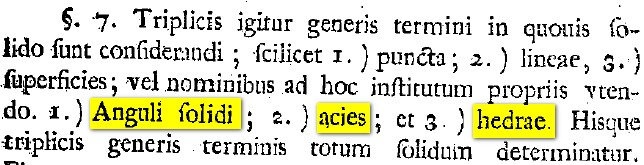Figure 1. V, E, F defined.

Euler also repeatedly refers to two additional quantities. The first of these is anguli planius, the plane angles of the polygons that constitute the faces of a given polyhedron. For example, a square consists of four plane angles; at each vertex of a cube, three squares meet. Therefore, since a cube has eight vertices, it contains a total of of twenty-four plane angles. The second quantity that appears is laternum, or sides of polygons. Using the example of a cube again: a cube has six faces that are squares, each of which has four sides. Therefore we would say that a cube has twenty-four laternum; this is different from the number of edges, which is twelve. As the reader has possibly discovered, every edge is the intersection of two polygonal faces along a single side, and thus in general, the number of edges is half the total number of sides of all faces. This fact is actually Euler's first Proposition, and will be discussed in detail later in our paper. Euler never assigns letters to represent the number of anguli planius or number of laternum, so to facilitate classroom discussion, you may want to declare that these quantities be denoted by P and L. Another early observation of Euler is that P = L, a fact that he will use repeatedly in his subsequent propositions.

Since these terms are new to Euler's audience (as well as our students), the pedagogically sound next step is to look at an example. Therefore, in paragraph 8, Euler discusses a cuneiforme, or wedge-shape. (The word cuneiforme also refers to the wedge-shaped style of writing used by the ancient Sumerians and Babylonians.) Euler includes a picture of a wedge, labels the vertices, and explicitly lists all six vertices, nine edges, and five faces.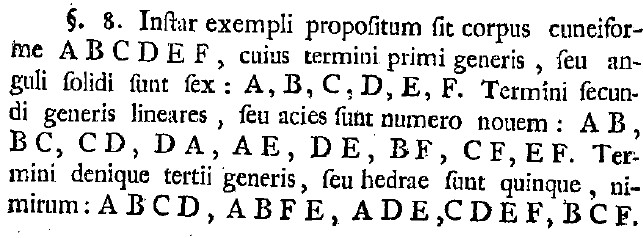Figure 2. Euler's wedge-shaped example.

In paragraph 9, Euler mentions the five regular polyhedra, which we commonly call the Platonic solids today. (Note that the "hexahedron" refers to the cube.)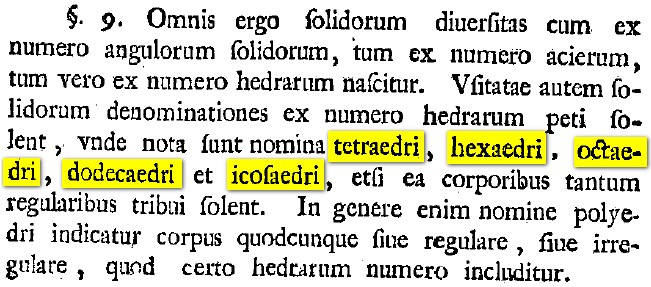Figure 3. Regular polyhedra are mentioned.

In paragraph 10, Euler proposes a general naming system for polyhedra based on the number of vertices and faces, not dissimilar from the genus-species system used to classify living organisms today. True to his style, after discussing generalities, Euler proceeds to give specific examples of the names of some common solids under his new system in paragraph 11. In particular, the wedge-shape previously considered shall be called a pentaedrum hexagonum, as it has five faces and six vertices. The other examples of solids mentioned by Euler are a triangular pyramid, a triangular prism, and a parallelepiped. It might aid student comprehension to review the Greek names for the numbers from one to twenty at this point.

These three sections naturally lend themselves to a classroom discussion. What would Euler call a pentagonal prism? What would a hexagonum octaedrum be called today? Students should be given some time to become acquainted with the geometric terms before moving on; Euler's presentation style is very conducive to this. Depending on the depth to which a teacher wishes to cover this topic, there are deeper questions that can be explored at this junction. What are the advantages and disadvantages to Euler's naming system? Is it possible that two different solids would have the same name under this system? What do we mean by "different" in this sense? For example, should we consider a cube and a truncated square pyramid as "different"?

# Investigating Euler's Polyhedral Formula Using Original Sources - Euler's Proposition 1

Author(s):

Next we shall consider some of Euler's results -- propositions and corollaries -- in the original Latin! In particular, we hope to convey to the reader that the language is not an obstacle to be overcome but a part of the experience, and something to be enjoyed along the way.

Proposition 1 (paragraph 21) says (in part):

In quouis solido numeris omnium acierum est semissis numeri omnium angulorum planorum. . . .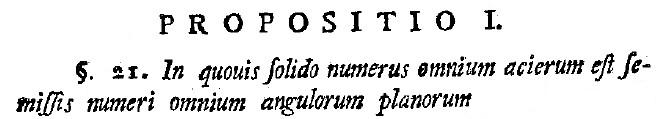Figure 4. Euler's Proposition 1.

Again, we emphasize that the reader knows more Latin than they suspect. Some of these words are already familiar: solido means "solid", acierum means "edges", angulorum planorum means "plane edges. It is a fair guess that numeris means "number". The word omnium is a bit harder, but the root, omni, appears in many words: omnipotent (all-powerful), omniscient (all-knowing), etc. Thus we will guess (at least for the purposes of a first pass) that omnium means "all". The word semissis also appears intimidating, but the root, semi, is also common in English, and we will translate it as "half". The word quouis we check in a Latin-to-English dictionary and find that it refers to an indefinite object (whoever/whichever/whatever); based on context, we'll try "whatever". Finally, we try the smaller words. A glance at our dictionary reveals that in is a preposition which could have various meanings; here we will try using "in" as our translation. The word est will be recognized by those familiar with French as a conjugation of that all-important verb "to be"; we will translate est as "is". Thus our amateur translation of Proposition 1 is:

In whatever solid number all edges is half number all plane angles. . . .

Making a second pass and inserting a few articles from English will increase the readability:

In whatever solid the number of all edges is half the number of all plane angles. . . .

Mathematically speaking, this is the formula:

$$E = \frac{1}{2}P$$

We have now uncovered the first formula in the paper! Of course, the next natural question to ask is: Why is this true? Depending on one's goals, one could use guided discovery ("Since E is an integer, what must be true about P? It must be—it is necessary that it is—divisible by two, or in other words, P is even!"), students could reason this out without reading further, students could translate Euler's explanation (as Euler calls it, the Demonstratio), or groups could work on these tasks in parallel.

Continuing on, let us examine Corollary 2 (paragraph 23) of this Proposition:

Si igitur omnes hedrae ambitum solidi cuiuspiam constituentes fuerint triangula, earum numerus necessario erit par. . . .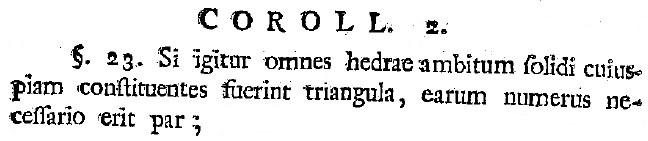Figure 5. Euler's Corollary 2 to Proposition 1.

There are some familiar words here, and a few new unfamiliar ones. Euler keeps the total amount of vocabulary required for reading his papers relatively low, so the translations will eventually become easier as we progress through the paper.

Looking up the word si in a dictionary, we translate it as "if"; we translate igitur as "therefore". The word omnes appears similar in root to omnium from the previous Proposition, so we translate it as "all" once again. Hedrae will still mean "faces". The word ambitum appears to have many possible translations, so we will choose one possible definition that seems general for now and try to find a better choice of meaning later once we have a better idea of the context. We will choose "around" for ambitum. Solidi will still mean "solid". Cuiuspiam appears to mean "any". Constituentes appears similar in root to the English word "constitute". Fuerint is another form of the verb "to be", which we loosely translate as "is". We can guess that triangula means "triangle(s)" or "triangular"; again, the form we need will be more clear once we have an idea of the context. Looking up earum, we find the meaning "they". Numerus we will guess as "number" again, and necessario looks a lot like the word "necessary", so that will be our guess for now. Erit is yet another form of "to be", and again we will translate it as "is" until it is clear what conjugation we require. Finally, par is an interesting word with many definitions, such as "mate", "spouse", or "partner". Reading down the list of possibilities in our dictionary, we find a particularly mathematical translation: "even" (as in "even number"), which we will use as our guess for now. Upon reflection, we note that par is the root of "parity".

Thus, our very rough first translation is:

If therefore all faces around solid any constitute is triangle(s), they number necessary is even. . . .

This translation isn't perfect, but remember, our main goal is to locate and understand the mathematical content. Euler appears to be saying that:

If all faces around any solid are made from triangles, their (total) number is necessarily even. . . .

The word "therefore" occurs at the beginning of Euler's sentence since this is a Corollary; that this result appears as a Corollary indicates that the main part of the argument will follow from the truth of the Proposition previously mentioned. "The Proposition is true, therefore the Corollary is true" is a standard and well-known presentation format to today's mathematicians; however, this dependence is not obvious to students and hence appropriately emphasized by inclusion of the word "therefore".

Now, assuming that we are comfortable with the mathematical content of this paragraph, let us investigate it in more detail. The proof is another good topic for discussion or guided discovery... why is this result true? Since this is a Corollary, as mentioned above, it should depend on the Proposition, which was

$$E = \frac{1}{2}P$$

If the faces of a solid are all triangles, what do we know about the value of P? What do we know about the value of E when the number of triangles is odd? Try some examples. What about the value of E when the number of triangles is even? Again, Euler has a nice, gentle progression of ideas suitable for exploration in the classroom.

# Investigating Euler's Polyhedral Formula Using Original Sources - Euler's Proposition 2

Author(s):

As we progress through the paper, our vocabulary becomes larger and the propositions become easier to comprehend. Euler's second Proposition (paragraph 26) is:

Numerus omnium angulorum planorum vel aequalis est vel maior numero omnium hedrarum ter sumto.
Vel numerus angulorum planorum nunquam minor esse potest, quam triplum numeri hedrarum ambitum solidi cuiusquam.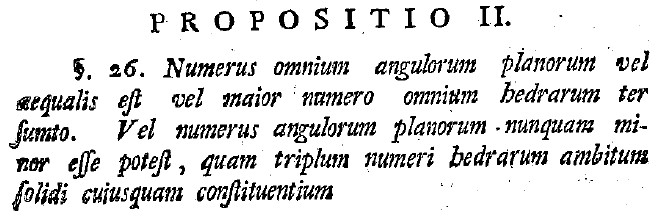Figure 6. Euler's Proposition 2.

Many of these words are familiar by now; we discuss those which are new. The word vel means "or"; the construction "vel . . . vel" means "either . . . or". We may guess that aequalis means "equal(s)". The word maior looks unfamiliar, but we may try swapping the "i" with a "j" as described earlier. Major we will translate as "greater". The word ter is the root of words such as "tertiary"; we will thus translate ter as "three". Sumto appears to have sum as a root; this might mean "sum" or "addition". In parallel with our translation of major above, we will translate minor as "lesser". Using our Latin-to-English dictionary, we guess that esse will mean "is", potest will mean "possible", quam could mean "who", "what", or "which", and nunquam could mean "at no time", "never", or "in no circumstances". It is a reasonable guess that triplum means "triple". And so our rough first translation becomes:

Number all plane angles either equal is or greater number all faces three sum.
Or number plane angles never lesser is possible, which triple number faces around solid any.

Gently rearranging words and using articles, our attempt at translation yields:

The number of all the plane angles is either equal to or greater than thrice (three times) the number that is the sum of all the faces.
The number of plane angles can never possibly be less than triple the number of faces around any solid.

Mathematically speaking, this proposition states (in modern notation) that:

Either $$P = 3F$$ or $$P > 3F$$.
It is never the case that $$P < 3F$$.

This is slightly more complicated than the last proposition. Students could find the proof using guided discovery or by translating Euler's line of reasoning -- whatever method works best for the course and students at hand. Again, it might be helpful for students to see examples of solids for which the equality holds and for which the inequality holds. Teachers could prepare a batch of examples for use in the classroom, or see what Euler has to say in Corollary 1 to this proposition:

Si ergo omnes hedrae fuerint triangulares, numerus angulorum planorum aequalis erit triplo numeri hedrarum. . . .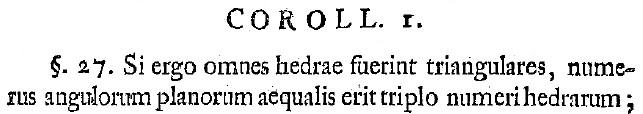Figure 7. Euler's Corollary 1 to Proposition 2.

The only new word here is ergo, which we may recognize as part of the famous quote "cogito ergo sum"—"I think, therefore I am". Thus we translate ergo as "therefore", and our rough first translation is:

If therefore all faces is triangle(s), number plane angles equal is triple number faces. . . .

A slight revision would yield:

Therefore, if all faces are triangles, the number of plane angles is equal to triple the number of faces. . . .

Again, the word "therefore" arises because a corollary follows from the proposition appearing before it. Euler has given us an example of when equality holds in Proposition 2. Why not ask students to check it for the tetrahedron, octahedron, and dodecahedron?

Let us examine the second corollary of Proposition 2.

In solido igitur quocunque, fi numeris hedrarum ponatur = H, et numerus acierum = A,
quia numeris angulorum planorum =
2A, erit vel 2A = 3H vel 2A > 3H.
Impossibile ergo est, ut sit
2A < 3H.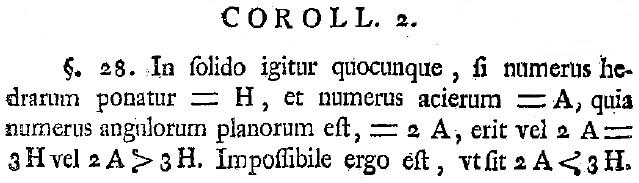Figure 8. Euler's Corollary 2 to Proposition 2.

The further we progress, the easier the translations become! New words appearing here include ponatur, which could mean "put" or "set" (we will use the latter), quia, which we look up and find means "because", and ut, which we will translate as "while". The rough translation is:

In solid therefore whatever, if number faces set = $$H$$, and number edges = $$A$$,
because number plane angles = $$2A$$, is either $$2A = 3H$$ or $$2A > 3H$$.
Impossible therefore is, while is $$2A < 3H$$.

Cleaning up the translation (and using modern notation), we have:

Therefore, in whatever solid, if the number of faces is set equal to $$F$$, and the number of edges equals $$E$$,
because the number of plane angles equals $$2E$$, it is either [the case that] $$2E = 3F$$ or $$2E > 3F$$.
While therefore it is impossible that $$2E < 3F$$.

In short:

Either $$2E = 3F$$ or $$2E > 3F$$; It is never the case that $$2E < 3F$$.

The proof of this is easy: we are doing a simple substitution, combining Proposition 1 and Proposition 2. This would be such a small step that most authors would omit it, much less set it apart as a corollary by itself. Euler, however, is not most authors. He sets a good pace for students of mathematics, from any century, seeing this for the first time.

Proposition 3 is similar to Proposition 2, and therefore we omit a discussion of it here, although it is still important and should be discussed as it will be important later. Proposition 3, in modern notation, states that

Either $$P = 3V$$ or $$P > 3V$$; It is never the case that $$P < 3V$$.

The second corollary to this proposition states that:

Either $$2E = 3V$$ or $$2E > 3V$$; It is never the case that $$2E < 3V$$.

# Investigating Euler's Polyhedral Formula Using Original Sources - Euler's Polyhedral Formula

Author(s):

Proposition 4 is the most famous one; we have arrived at "Euler's Polyhedral Formula".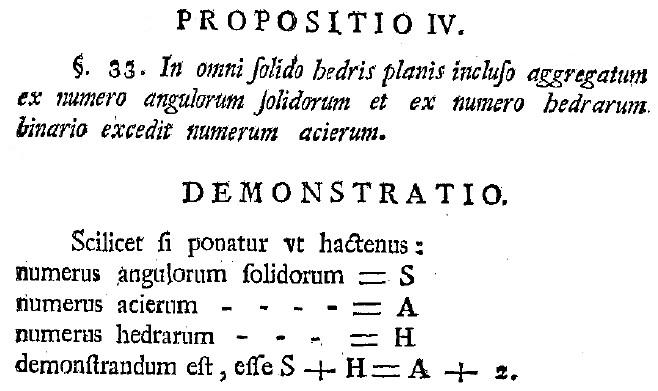Figure 9. Euler's Polyhedral Formula.

After reminding us what the variables stand for (a good mathematical practice in general!), Euler writes the result

$$S + H = A + 2$$,

which we would write in modern notation as

$$V + F = E + 2$$.

Euler admits right away that he does not have a firm proof of this theorem, but in an effort to convince the reader, he works through eight classes of examples. He refers to figures at the back of the article, but in reality each picture is just meant as an example of the class for which Euler writes a proof. In the first four classes of examples, Euler proves that this formula holds for pyramids, wedges, prisms, and cupola-like objects.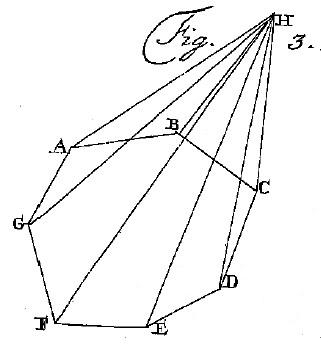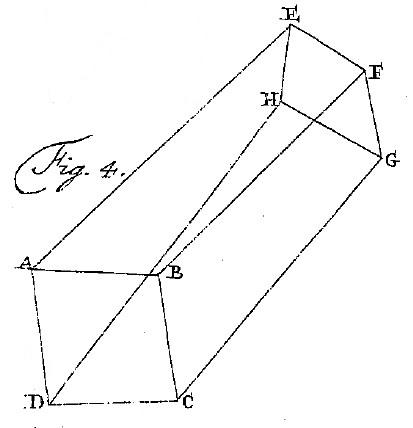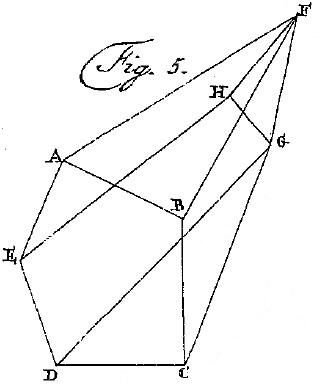Figure 10. Pyramids, Wedges, Prisms, and Cupola-like Solids.

These first four classes actually form an elegant progression of solids of increasingly complexity:

• Pyramids have a base that is an n-gon, all of whose vertices are connected via edges to a single point;
• Wedges have an n-gon base with vertices connected to one of the two endpoints of a line segment;
• Prisms have an n-gon base with vertices connected to the vertices of another n-gon;
• Cupola-like solids have an n-gon base with vertices connected to the vertices of an m-gon, where m is any positive integer except 1, 2, or n (as these cases were previously covered).

Euler concludes his demonstration by verifying the formula holds for the Platonic solids.

Working through these verifications for specific cases (such as finding the values of V, E, and F, for the pentagonal prism and checking that the formula holds) and for general cases (such as for an n-gonal prisms, finding values for V, E, and F in terms of n, and checking that the formula holds) is invaluable practice for students.

There is also an important discussion to be had, centered on a pair of questions:

1. Are you convinced that Euler's formula is true?
2. Did Euler prove that the polyhedral formula is true?

After gathering answers to these two questions, students can explore how the answers to these two questions are related. For example, ask students: If you are convinced, is it a proof? What should be the role of a proof, besides convincing the reader? What if the proof is too complicated and does not convey understanding—is a series of examples better? Can a series of examples be converted into a proof? There is a wealth of good discussion material to be had here.

Also interesting are the three corollaries to Proposition 4. They are: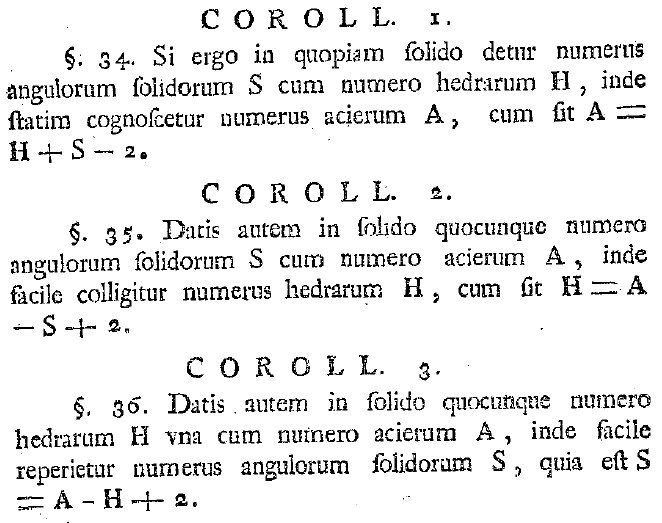Figure 11. Polyhedral Formula Corollaries.

In short, using modern terminology:

Corollary 1: $$E = F + V - 2$$
Corollary 2: $$F = E - V + 2$$
Corollary 3: $$V = E - F + 2$$

What is particularly striking about the four different formulations of the polyhedral formula is that among them, we do not find the formula written in the form that it is typically found today:

$$V - E + F = 2$$

Reading "between the lines", we hypothesize that this is due to a shift in the way we interpret the significance of these equations. To Euler, perhaps, these equations provide a method to solve for any one of these three variables if given the values of the other two. However, a more modern interpretation of the significance of this equation is that the quantity $$V - E + F$$ is the same (it is equal to 2) for any solid in the classes that Euler considered; the number 2 is a characteristic (or an invariant) for these classes of solids. Other solids (such as tori) will result in a different characteristic number at the end of such a formula.

# Investigating Euler's Polyhedral Formula Using Original Sources - Additional Results

Author(s):

There are many, many more results in this paper of Euler than can be addressed in this article. We will briefly mention a few additional results.

Proposition 5 (paragraph 37) states:

It is not possible that $$E + 6 > 3F$$ or $$E + 6 > 3V$$.

Proposition 6 (paragraph 42) states:

It is not possible that $$F + 4 > 2V$$ or $$V + 4 > 2F$$.

Euler then combines many of his propositions in an exciting way. From Proposition 2, Corollary 2, we know that $$2E ≥ 3F$$, or equivalently, $$\frac{3}{2}F ≤ E$$. From Proposition 5, we know that it is not possible that $$E + 6 > 3F$$, or equivalently, $$E ≤ 3F - 6$$. Therefore, if we know the number of faces, $$F$$, of a polyhedron, we now know upper and lower bounds on the number of edges, $$E$$:

$$\frac{3}{2}F ≤ E ≤ 3F - 6$$.

From Proposition 6, we know that $$F + 4 ≤ 2V$$ and $$V + 4 ≤ 2F$$, or equivalently, that $$\frac{1}{2}F + 2 ≤ V$$ and $$V ≤ 2F - 4$$. Therefore, if we know the number of faces, $$F$$, of a polyhedron, we now know upper and lower bounds on the number of vertices, $$V$$:

$$\frac{1}{2}F + 2 ≤ V ≤ 2F - 4$$.

In Proposition 6, Corollary 5 (paragraph 47), Euler uses all of these formulas together and classifies all of the different possible solids by number of faces, vertices, and edges. He produces the following table: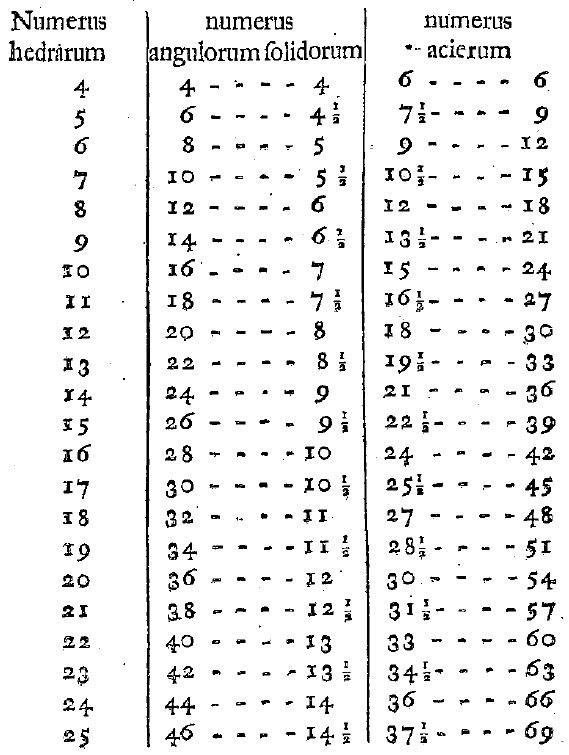Figure 12. Bounds on Vertices and Edges for Given Number of Faces.

In the first column are different possible values for the number of faces of a polyhedron ranging from $$F = 4$$ to $$F = 25$$. For each value of $$F$$ there is a range of possible values for $$V$$ and $$E$$, and these ranges are the contents of the second and third columns of the table, respectively.

This table can be used in a classroom lesson in a variety of ways. Students can be given the equations above to re-create the table for themselves or simply to extend the table (perhaps for solids with up to $$30$$ faces), or alternatively students could be given the table and asked to locate the equations in Euler's paper that are give rise to these values. Students could be asked to build a similar table: one that gives ranges for the values of $$F$$ and $$V$$ given values of $$E$$; this is Euler's Corollary 7 to this proposition (paragraph 49); Euler tackles the cases from $$E = 6$$ to $$E = 60$$.

Propositions 7, 8, and 9 are mentioned in Dr. Sandifer's column in How Euler Did It, and so we will not discuss them here.

# Investigating Euler's Polyhedral Formula Using Original Sources - Euler's Grand Problem

Author(s):

We would be remiss if we did not mention the grand finale to this wonderful work of Euler. In paragraph 59, Euler presents a grand problem: to classify all solids.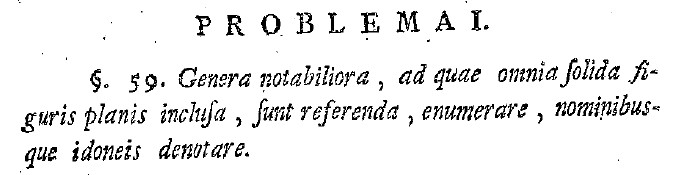Figure 13. Euler's Grand Problem.

This requires results from throughout the paper. This is a good final experience for students as well.

Thanks to the groundwork laid by Euler, this is a relatively straightforward question. First, he starts by choosing a value for V. Using the inequalities previously developed, there is a range of possible values for F. For each possible value of F, use the polyhedral formula (Proposition 4) to find the corresponding value of E. Euler lists the possibilities in a table, for solids with up to 10 vertices, along with their names using his nomenclature.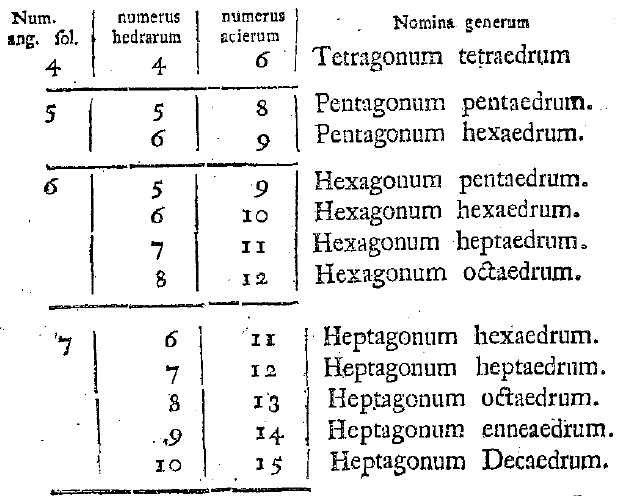Figure 14. Partial Solution to the Grand Problem.

This naturally lends itself to a number of activities:

• Create or extend the table given by Euler.
• Draw diagrams of the solids mentioned in the table. The shape of the faces of a given solid—triangular, square, etc.—is not obvious, given the tabulated data.
• Match Euler's names with modern names. For example, among the first seven entries in this table, you will find what we refer to today as a tetrahedron, a square pyramid, a pentagonal pyramid, a triangular dipyramid, an octahedron, a triangular prism, and one solid without a standard name.

This concludes our overview of the many interesting results to be found in this paper, and we hope we have inspired the reader with some ideas for using this original paper of Euler in the classroom.

In closing, we paraphrase a famous saying:

Read Euler, Read Euler; he is an author for us all . . . even our students.

Author(s):